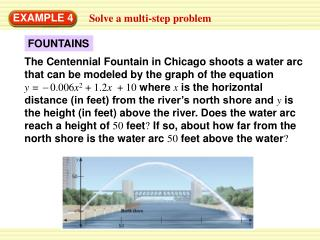Download PresentationFOUNTAINS

# FOUNTAINS

Télécharger la présentation## FOUNTAINS

- - - - - - - - - - - - - - - - - - - - - - - - - - - E N D - - - - - - - - - - - - - - - - - - - - - - - - - - -
##### Presentation Transcript

1. EXAMPLE 4 Solve a multi-step problem FOUNTAINS The Centennial Fountain in Chicago shoots a water arc that can be modeled by the graph of the equation y = 0.006x2 + 1.2x + 10 where xis the horizontal distance (in feet) from the river’s north shore and yis the height (in feet) above the river. Does the water arc reach a height of 50 feet? If so, about how far from the north shore is the water arc 50 feet above the water?

2. y 0.006x2 1.2x 10 = – + + = – + 50 0.006x2 1.2x 10 + = – – 0 0.006x2 1.2x 40 + EXAMPLE 4 Solve a multi-step problem SOLUTION STEP 1 Write a quadratic equation. You want to know whether the water arc reaches a height of 50 feet, so let y = 50. Then write the quadratic equation in standard form. Write given equation. Substitute 50 for y. Subtract 50 from each side.

3. Find the value of the discriminant of 0 = –0.006x2 + 1.2x – 40. – b2 4ac = (1.2)2 4(–0.006)(– 40) – a=– 0.006, b = 1.2, c = – 40 = 0.48 Simplify. EXAMPLE 4 Solve a multi-step problem STEP 2 STEP 3 Interpret the discriminant. Because the discriminant is positive, the equation has two solutions. So, the water arc reaches a height of 50 feet at two points on the water arc.

4. 0 = –0.006x2 + 1.2x – 40 x 42 or x 158  b 2 4ac – – b ± x = 2a Solve the equation to find the distance from the north shore to where the water arc is 50 feet above the water.  0.48 1.2 ± – = 2(–0.006) EXAMPLE 4 Solve a multi-step problem STEP 4 Quadratic formula Substitute values in the quadratic formula. Use a calculator.

5. ANSWER The water arc is 50 feet above the water about 42 feet from the north shore and about 158 feet from the north shore. EXAMPLE 4 Solve a multi-step problem

6. ANSWER Yes; 100 ft for Example 4 GUIDED PRACTICE 7.WHAT IF?In Example 4, does the water arc reach a height of 70 feet? If so, about how far from the north shore is the water arc 70 feet above the water?